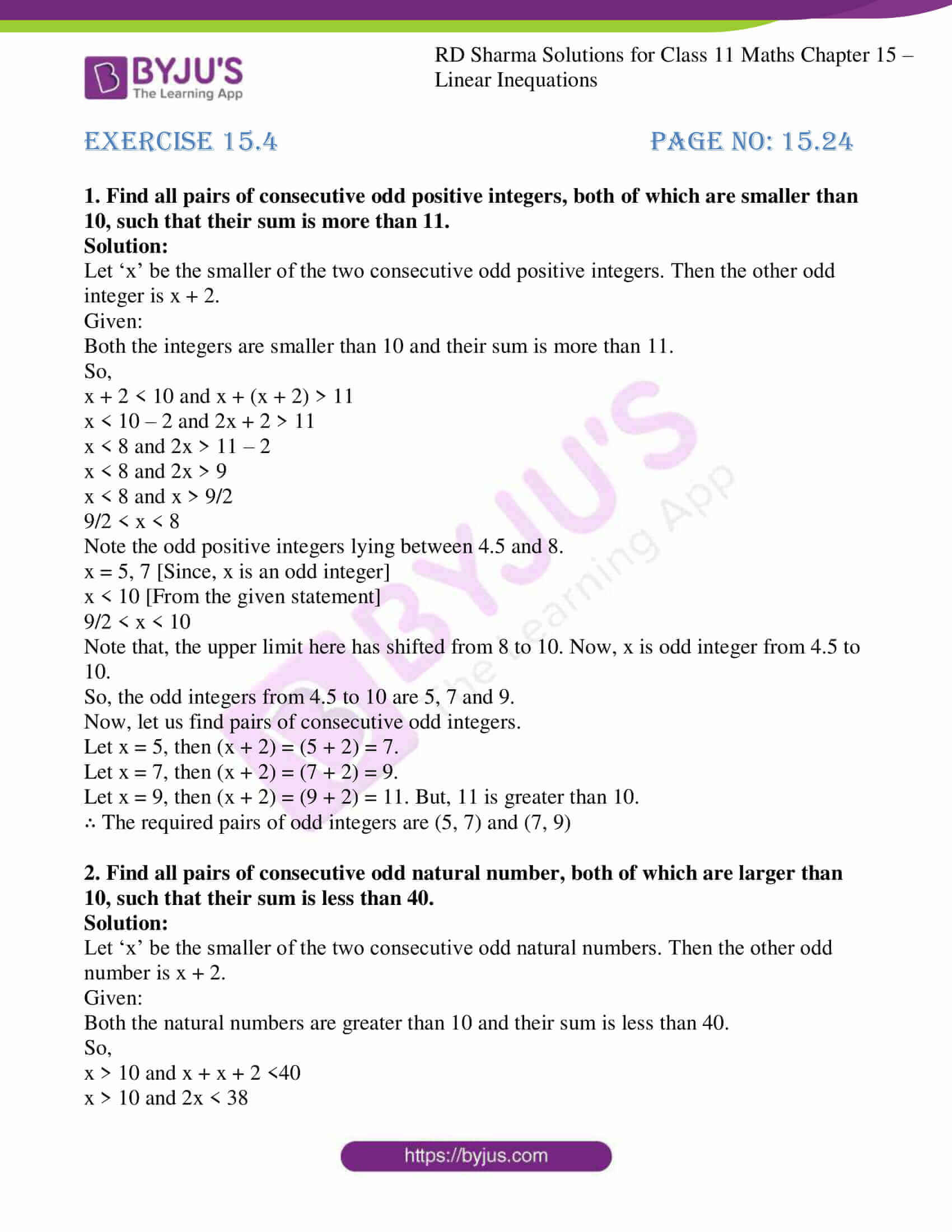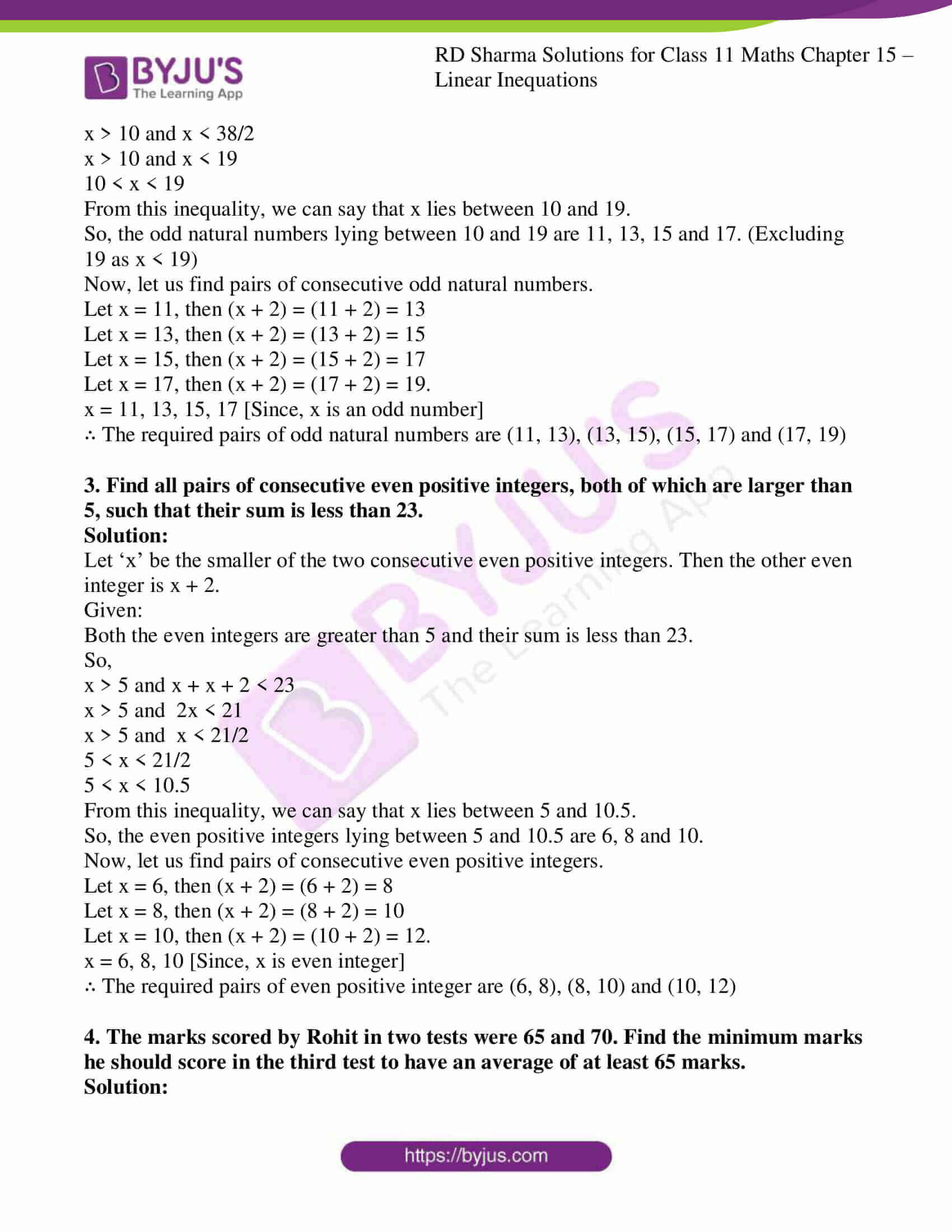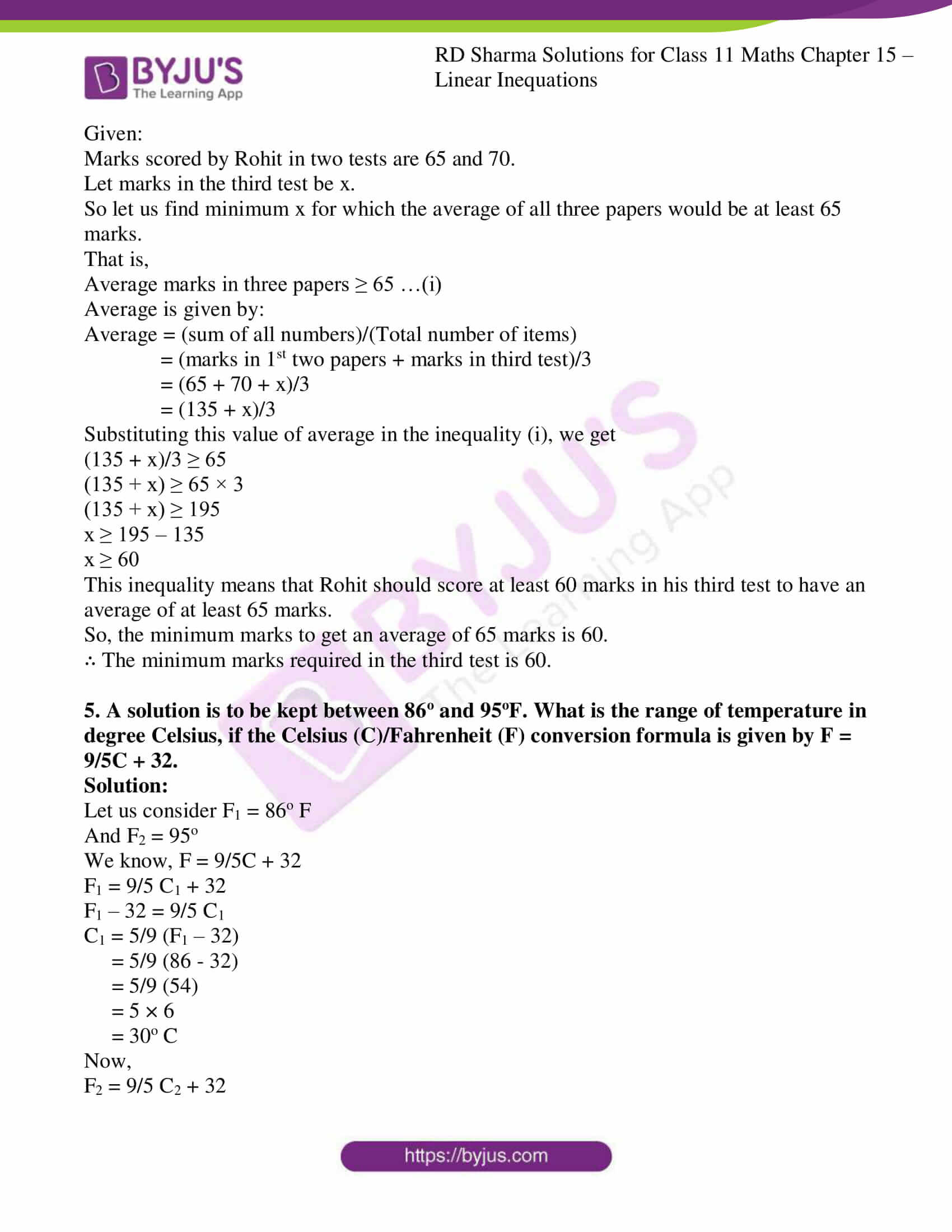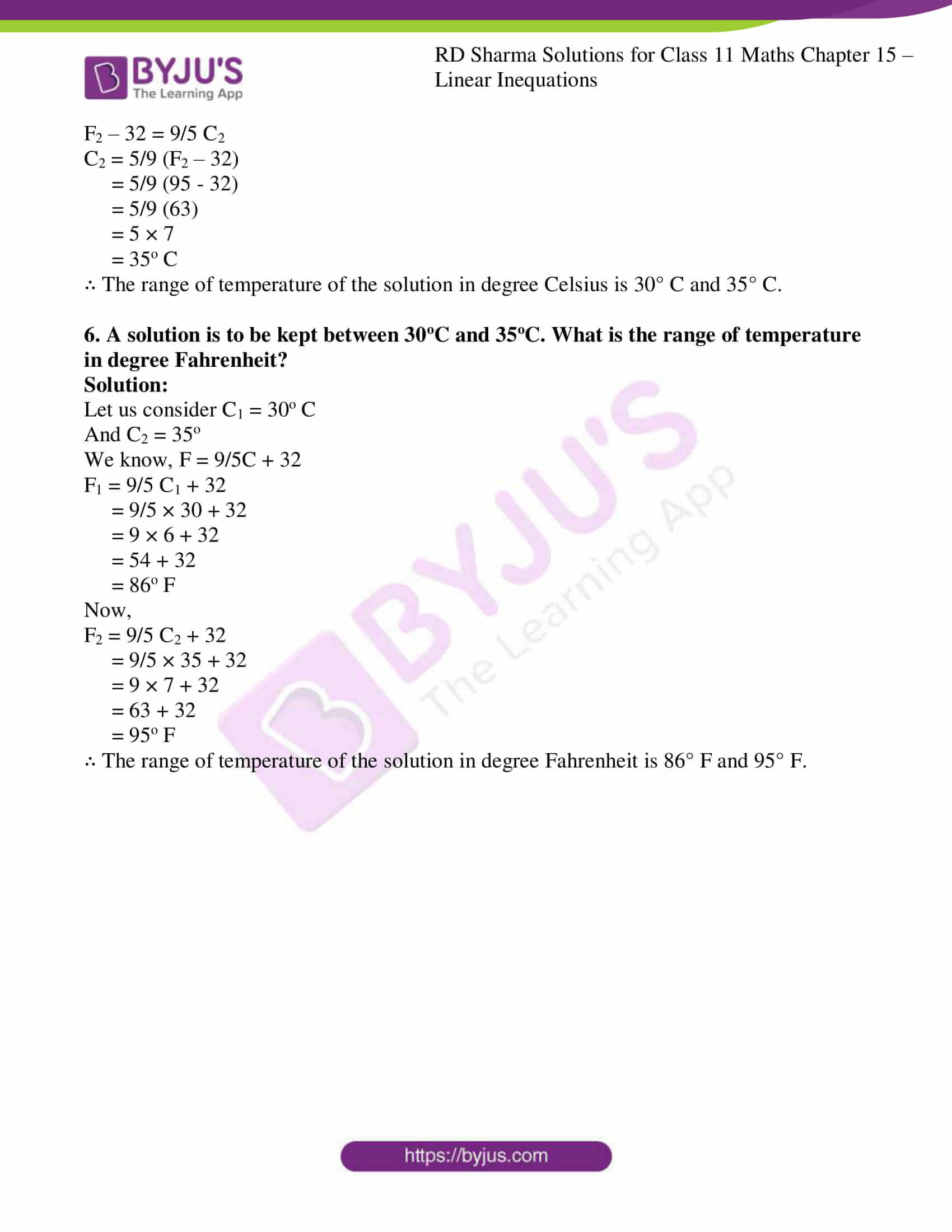# RD Sharma Solutions for Class 11 Chapter 15 - Linear Inequations Exercise 15.4

In this section, we shall utilize the knowledge of solving linear inequations in one variable in solving different problems from various fields such as science, engineering, economics etc. Our experts have solved the RD Sharma solutions to ensure that students gain in-depth knowledge about the concepts by practising the solutions. Students are advised to practice the solutions diligently so that they can attain the desired scores. Students can refer and download RD Sharma Class 11 Maths Solutions from the links provided below.

## Download the pdf of RD Sharma Solutions for Class 11 Maths Exercise 15.4 Chapter 15 – Linear Inequations### Access answers to RD Sharma Solutions for Class 11 Maths Exercise 15.4 Chapter 15 – Linear Inequations

1. Find all pairs of consecutive odd positive integers, both of which are smaller than 10, such that their sum is more than 11.

Solution:

Let ‘x’ be the smaller of the two consecutive odd positive integers. Then the other odd integer is x + 2.

Given:

Both the integers are smaller than 10 and their sum is more than 11.

So,

x + 2 < 10 and x + (x + 2) > 11

x < 10 – 2 and 2x + 2 > 11

x < 8 and 2x > 11 – 2

x < 8 and 2x > 9

x < 8 and x > 9/2

9/2 < x < 8

Note the odd positive integers lying between 4.5 and 8.

x = 5, 7 [Since, x is an odd integer]

x < 10 [From the given statement]

9/2 < x < 10

Note that, the upper limit here has shifted from 8 to 10. Now, x is odd integer from 4.5 to 10.

So, the odd integers from 4.5 to 10 are 5, 7 and 9.

Now, let us find pairs of consecutive odd integers.

Let x = 5, then (x + 2) = (5 + 2) = 7.

Let x = 7, then (x + 2) = (7 + 2) = 9.

Let x = 9, then (x + 2) = (9 + 2) = 11. But, 11 is greater than 10.

∴ The required pairs of odd integers are (5, 7) and (7, 9)

2. Find all pairs of consecutive odd natural number, both of which are larger than 10, such that their sum is less than 40.

Solution:

Let ‘x’ be the smaller of the two consecutive odd natural numbers. Then the other odd number is x + 2.

Given:

Both the natural numbers are greater than 10 and their sum is less than 40.

So,

x > 10 and x + x + 2 <40

x > 10 and 2x < 38

x > 10 and x < 38/2

x > 10 and x < 19

10 < x < 19

From this inequality, we can say that x lies between 10 and 19.

So, the odd natural numbers lying between 10 and 19 are 11, 13, 15 and 17. (Excluding 19 as x < 19)

Now, let us find pairs of consecutive odd natural numbers.

Let x = 11, then (x + 2) = (11 + 2) = 13

Let x = 13, then (x + 2) = (13 + 2) = 15

Let x = 15, then (x + 2) = (15 + 2) = 17

Let x = 17, then (x + 2) = (17 + 2) = 19.

x = 11, 13, 15, 17 [Since, x is an odd number]

∴ The required pairs of odd natural numbers are (11, 13), (13, 15), (15, 17) and (17, 19)

3. Find all pairs of consecutive even positive integers, both of which are larger than 5, such that their sum is less than 23.

Solution:

Let ‘x’ be the smaller of the two consecutive even positive integers. Then the other even integer is x + 2.

Given:

Both the even integers are greater than 5 and their sum is less than 23.

So,

x > 5 and x + x + 2 < 23

x > 5 and 2x < 21

x > 5 and x < 21/2

5 < x < 21/2

5 < x < 10.5

From this inequality, we can say that x lies between 5 and 10.5.

So, the even positive integers lying between 5 and 10.5 are 6, 8 and 10.

Now, let us find pairs of consecutive even positive integers.

Let x = 6, then (x + 2) = (6 + 2) = 8

Let x = 8, then (x + 2) = (8 + 2) = 10

Let x = 10, then (x + 2) = (10 + 2) = 12.

x = 6, 8, 10 [Since, x is even integer]

∴ The required pairs of even positive integer are (6, 8), (8, 10) and (10, 12)

4. The marks scored by Rohit in two tests were 65 and 70. Find the minimum marks he should score in the third test to have an average of at least 65 marks.

Solution:

Given:

Marks scored by Rohit in two tests are 65 and 70.

Let marks in the third test be x.

So let us find minimum x for which the average of all three papers would be at least 65 marks.

That is,

Average marks in three papers ≥ 65 …(i)

Average is given by:

Average = (sum of all numbers)/(Total number of items)

= (marks in 1st two papers + marks in third test)/3

= (65 + 70 + x)/3

= (135 + x)/3

Substituting this value of average in the inequality (i), we get

(135 + x)/3 ≥ 65

(135 + x) ≥ 65 × 3

(135 + x) ≥ 195

x ≥ 195 – 135

x ≥ 60

This inequality means that Rohit should score at least 60 marks in his third test to have an average of at least 65 marks.

So, the minimum marks to get an average of 65 marks is 60.

∴ The minimum marks required in the third test is 60.

5. A solution is to be kept between 86o and 95oF. What is the range of temperature in degree Celsius, if the Celsius (C)/Fahrenheit (F) conversion formula is given by F = 9/5C + 32.

Solution:

Let us consider F1 = 86o F

And F2 = 95o

We know, F = 9/5C + 32

F1 = 9/5 C1 + 32

F1 – 32 = 9/5 C1

C1 = 5/9 (F1 – 32)

= 5/9 (86 – 32)

= 5/9 (54)

= 5 × 6

= 30o C

Now,

F2 = 9/5 C2 + 32

F2 – 32 = 9/5 C2

C2 = 5/9 (F2 – 32)

= 5/9 (95 – 32)

= 5/9 (63)

= 5 × 7

= 35o C

∴ The range of temperature of the solution in degree Celsius is 30° C and 35° C.

6. A solution is to be kept between 30oC and 35oC. What is the range of temperature in degree Fahrenheit?

Solution:

Let us consider C1 = 30o C

And C2 = 35o

We know, F = 9/5C + 32

F1 = 9/5 C1 + 32

= 9/5 × 30 + 32

= 9 × 6 + 32

= 54 + 32

= 86o F

Now,

F2 = 9/5 C2 + 32

= 9/5 × 35 + 32

= 9 × 7 + 32

= 63 + 32

= 95o F

∴ The range of temperature of the solution in degree Fahrenheit is 86° F and 95° F.